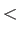### Keyword PRINT

Optional printing can be activated with this keyword.
Options:
 AUXIS Print auxiliary function table. BASIS Print molecular orbitals in long format. C6 Print Ccoefficients. CGTO Print GTO contraction table. CIS Print CIS three-center electron repulsion integral batching. CON Print MD constraints. COORD Print primitive internal coordinates. CP Print critical points during Bader population analysis. DE2 Print Hessian matrix. DEBUG Generate debug output. ECP Print ECP table. EMBED Print embedding charges. ERIS Print three-center electron repulsion integrals. FIT Print preconditioned conjugate gradient cycles. FUKUI Print Fukui matrices. G Print auxiliary function Coulomb matrix. GRID Generate full grid output. GTO Print primitive GTO table. IRC Print verbose IRC output. KS Print Kohn-Sham and core Hamiltonian matrix. MAX=IntegerMaximum number of SCF cycles with print output. MCP Print MCP table. MD Print verbose MD simulation output. MOE Print molecular orbital energies and occupations. MOS Print molecular orbital energies, occupations and coefficients. NMR Print full NMR output. NOGEO Suppress geometry output. MAG Print full magnetizability output. NIA Print NIA CPKS cycles. NQR Print full NQR output. OPT Generate full optimization output. ORTHO Print orthogonalization matrix. P Print density matrix. POPAN Generate full population analysis output. R Print response matrix. RAM Print RAM allocation table. S Print overlap matrix. SADDLE Print verbose saddle-point interpolation output. SCF Print SCF cycles which are usually omitted during, for example, optimization and frequency analysis. SYMMETRY Generate full symmetry output. T Print kinetic energy matrix. TB Print tight-binding matrices. TD Print full TDDFT output. VERBOSE Print verbose information regarding the SVD procedure. XCE Generate full exchange-correlation energy output. XCV Generate full exchange-correlation potential output. XRAY Generate full x-ray spectroscopy output.
Description:
The MAX option specifies the number of SCF cycles with active print output. All matrices that are changing during the SCF (e.g. P, KS, MOS, etc.) can be printed for each cycle. The following example activates the printing of the density matrix in the first 10 SCF cycles:

``` PRINT MAX=10 P
```

The printing of molecular orbitals can be limited with the MOS option. The input MOS = <First> - <Last> specifies that only the MOs in the range from <First> through <Last> are printed. Here, <First> and <Last> refer to the (integer) indices of the molecular orbitals. With the MOE option, printing of the molecular orbital coefficients can be suppressed. This is recommended for larger basis sets if only MO energies are of interest. To print the orbital energies, occupation numbers, and coefficients of the orbitals 10 through 25 the following input can be used:

``` PRINT MOS=10-25
```

If only orbital energies and occupation numbers are of interest the input can be modified to:

``` PRINT MOE=10-25
```

In general, several print options such as MD, OPT, etc. will produce rather large outputs. In particular, the DEBUG option enables the printing of all matrices! Of course such an output is huge, even for small systems. Note also that the DEBUG option and some other PRINT options are only available in serial runs.